Tamilnadu State Board New Syllabus Samacheer Kalvi 12th Maths Guide Pdf Chapter 1 Applications of Matrices and Determinants Ex 1.4 Textbook Questions and Answers, Notes.

## Tamilnadu Samacheer Kalvi 12th Maths Solutions Chapter 1 Applications of Matrices and Determinants Ex 1.4

Question 1.
Solve the following systems of linear equations by Cramer’s rule:
(i) 5x – 2y + 16 = 0, x + 3y – 7 = 0
Solution: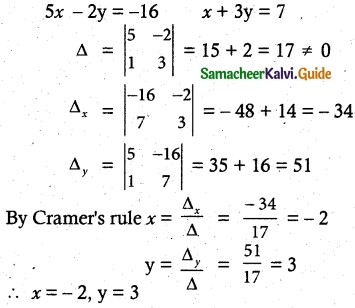(ii) $$\frac{3}{x}$$ + 2y =12, $$\frac{2}{x}$$ + 3y = 13
Solution:
Let $$\frac{1}{x}$$ = a
3a + 2b = 12
2a + 3b = 13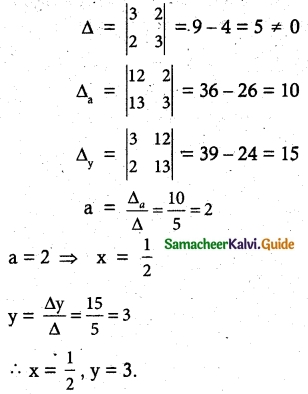(iii) 3x + 3y – z = 1 1, 2x – y + 2z = 9, 4x + 3y + 2z = 25
Solution:
Δ = $$\left| \begin{matrix} 3 & 3 & -1 \\ 2 & -1 & 2 \\ 4 & 3 & 2 \end{matrix} \right|$$
= 3(-2 – 6) -3 (4 – 8) -1(6 + 4)
= 3(-8) -3(-4) -1(10)
= -24 + 12 – 10 = -22 ≠ 0
Δx = $$\left| \begin{matrix} 11 & 3 & -1 \\ 9 & -1 & 2 \\ 25 & 3 & 2 \end{matrix} \right|$$
= 11 (-2 – 6) – 3(18 – 50) – 1(27 + 25)
= 11(-8) -3(32) -1(52)
= -88 + 96 – 52 = -44
Δy = $$\left| \begin{matrix} 3 & 11 & -1 \\ 2 & 9 & 2 \\ 4 & 25 & 2 \end{matrix} \right|$$
= 3(18 – 50) – 11(4 – 8) – 1(50 – 36)
= 3(32) -11(4) -1(14)
= -96 + 44 – 14 = -66
Δx = $$\left| \begin{matrix} 3 & 3 & 11 \\ 2 & -1 & 9 \\ 4 & 3 & 25 \end{matrix} \right|$$
= 3(-25 – 27) – 3(50 – 36) + 11(6 + 4)
= 3(-52) -3(14) + 11(10)
= -156 – 42 + 110
= -88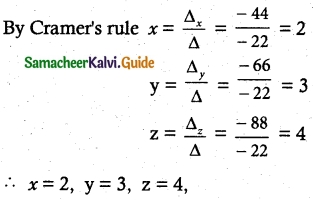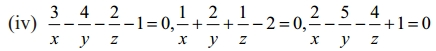Solution: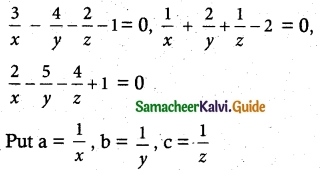3a – 4b – 2c = 1 ……….. (1)
a + 2b + c = 2 …………… (2)
2a – 5b – 4c = -1 ………….. (3)
Δ = $$\left| \begin{matrix} 3 & -4 & -2 \\ 1 & 2 & 1 \\ 2 & -5 & -4 \end{matrix} \right|$$
= 3(-8 + 5) + 4 (-4 – 2) – 2(-5 – 4)
= 3(-3) +4(-6) -2(-9)
= -9 – 24 + 18
= -15 ≠ 0
Δa = $$\left| \begin{matrix} 1 & -4 & -2 \\ 2 & 2 & 1 \\ -1 & -5 & -4 \end{matrix} \right|$$
= 1(-8 + 5) + 4(-8 + 1) – 2(-10 + 2)
= 1(-3) + 4(-7) – 2(-8)
= -3 – 28 + 16
= -15
Δb = $$\left| \begin{matrix} 3 & 1 & -2 \\ 1 & 2 & 1 \\ 2 & -1 & -4 \end{matrix} \right|$$
= 3(-8 + 1) – 1(-4 – 2) – 2(-1 – 4)
= 3(-7) -1(-6) – 2(-5)
= – 21 + 6 + 10 = -5
Δc = $$\left| \begin{matrix} 3 & -4 & 1 \\ 1 & 2 & 2 \\ 2 & -5 & -1 \end{matrix} \right|$$
= 3(-2 + 10) + 4(-1 – 4) + 1 (-5 – 4)
= 24 – 20 – 9 = -5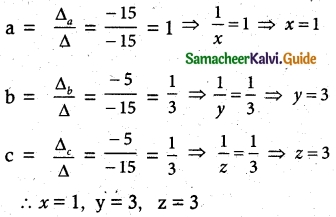Question 2.
In a competitive examination, one mark is awarded for every correct answer while $$\frac{1}{4}$$ mark is deducted for every wrong answer. A student answered 100 questions and got 80 marks. How many questions did he answer correctly? (Use Cramer’s rule to solve the problem).
solution:
No. of Questions answered = 100
Let the No. of questions answered correctly be x
and the No. of questions answered wrongly be y
Here, x + y = 100 and x – $$\frac { 1 }{ 4 }$$ y = 80
(i.e) x + y = 100 and 4x – y = 320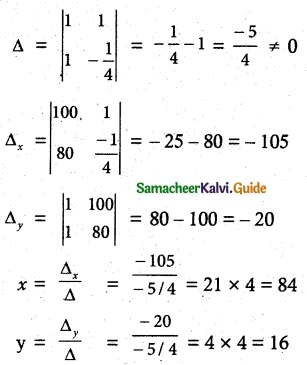correct questions = 84
wrong questions = 16.

Question 3.
A chemist has one solution which is 50% acid and another solution which is 25% acid. How much each should be mixed to make 10 litres of a 40% acid solution? (Use Cramer’s rule to solve the problem).
solution:
Let two solutions x and y
x + y = 10 …….. (1)
0.25 x + (0.50)y = (0.40) ……….. (2)
(2) × 100 ⇒ 25x + 50y = 400
(2) ÷ 5 ⇒ 5x + 10y = 80 …………. (3)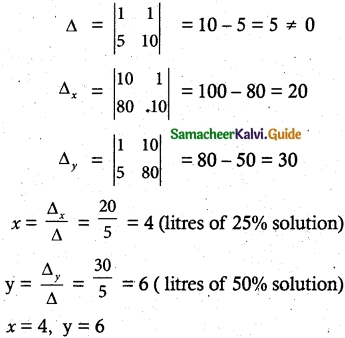Question 4.
A fish tank can be filled in 10 minutes using both pumps A and B simultaneously. However, pump B can pump water in or out at the same rate. If pump B is inadvertently run in reverse, then the tank will be filled in 30 minutes. How long would it take each pump to fill the tank by itself? (Use Cramer’s rule to solve the problems).
solution:
pump A fills $$(\frac {1}{x})^{th}$$ of the tank in 1 hour.
pump B fills $$(\frac {1}{y})^{th}$$ of the tank in 1 hour.
Both can filled $$(\frac {1}{10})^{th}$$ of the tank in 1 hour.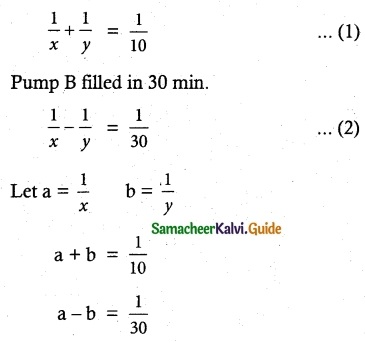using Cramer’s rule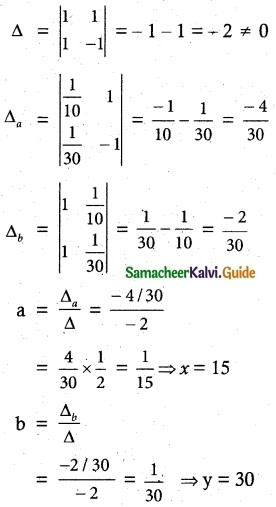Pump A takes 15 minutes
Pump B takes 30 minutesQuestion 5.
A family of 3 people went out for dinner in a restaurant. The cost of two dosai, three idlies and two vadais is Rs 150. The cost of the two dosai, two idlies and four vadais is Rs 200. The cost of five dosai, four idlies and two vadais is T 250. The family has Rs 350 in hand and they ate 3 dosai and six idlies and six vadais. Will they be able to manage to pay the bill within the amount they had?
solution:
Let the Cost of dosai, Idlies and vadais be x, y, z
2x + 3y + 2z = 150
2x + 2y + 4z = 200
5x + 4y + 2z = 250
Δ = $$\left| \begin{matrix} 2 & 3 & 2 \\ 2 & 2 & 4 \\ 5 & 4 & 2 \end{matrix} \right|$$
= 2(4 – 16) – 3(4 – 20) + 2(8 – 10)
= 2(-12) – 3(-16) + 2(-2)
= -24 + 48 – 4
= 20 ≠ 0
Δx = $$\left| \begin{matrix} 150 & 3 & 2 \\ 200 & 2 & 4 \\ 250 & 4 & 2 \end{matrix} \right|$$
= 150(4 – 16) – 3(400 – 1000) + 2(800 – 500)
= 150(-12) – 3(-600) + 2(300)
= -1800 + 1800 + 600
= 600
Δy = $$\left| \begin{matrix} 2 & 150 & 2 \\ 2 & 200 & 4 \\ 5 & 250 & 2 \end{matrix} \right|$$
= 2(400 – 1000) – 150(4 – 20) + 2(500 – 1000)
= 2(-600) – 150(-16) + 2(-500)
= -1200 + 2400 – 1000
= 200
Δz = $$\left| \begin{matrix} 2 & 3 & 150 \\ 2 & 2 & 200 \\ 5 & 4 & 250 \end{matrix} \right|$$
= 2(500 – 800) – 3(500 – 1000) + 150(8 – 10)
= 2(-300) – 3(-500) + 150(-2)
= -600 + 1500 – 300
= 600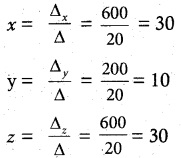x = Rs 30, y = Rs 10, z = Rs 30
There are 3 dosai, 6 idlies and 6 vadais
= 3x + 6y + 6z
= 3(30) + 6(10) + 6 (30)
= 90 + 60 + 180
= Rs. 330
They can eat within the amount.